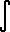#has-imagesxn dx = x(n+1) / (n+1) + C
If you want to change selection, open document below and click on "Move attachment"

Table of Integrals
Table of Integrals (Math | Calculus | Integrals | Table Of) Power of x. <span>x n dx = x (n+1) / (n+1) + C (n -1) Proof 1/x dx = ln|x| + C Exponential / Logarithmic &#13